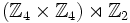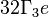# Wreath product of Z4 and Z2

View a complete list of particular groups (this is a very huge list!)[SHOW MORE]

## Definition

This group is defined as the wreath product of the cyclic group of order four and the cyclic group of order two. In other words, it is the semidirect product$(\Z_4 \times \Z_4) \rtimes \Z_2$ where the$\Z_2$ acts by coordinate exchange.

## Position in classifications

Type of classification Position/number in classification
GAP ID$(32,11)$, i.e.,$11^{th}$ among groups of order 32
Hall-Senior number 31 among groups of order 32
Hall-Senior symbol$32\Gamma_3e$

## Arithmetic functions

Want to compare and contrast arithmetic function values with other groups of the same order? Check out groups of order 32#Arithmetic functions

## Group properties

Want to compare and contrast group properties with other groups of the same order? Check out groups of order 32#Group properties
Property Satisfied? Explanation Comment
group of prime power order Yes
nilpotent group Yes prime power order implies nilpotent
supersolvable group Yes via nilpotent: finite nilpotent implies supersolvable
solvable group Yes via nilpotent: nilpotent implies solvable
abelian group No

## GAP implementation

### Group ID

This finite group has order 32 and has ID 11 among the groups of order 32 in GAP's SmallGroup library. For context, there are 51 groups of order 32. It can thus be defined using GAP's SmallGroup function as:

SmallGroup(32,11)

For instance, we can use the following assignment in GAP to create the group and name it$G$:

gap> G := SmallGroup(32,11);

Conversely, to check whether a given group$G$ is in fact the group we want, we can use GAP's IdGroup function:

IdGroup(G) = [32,11]

or just do:

IdGroup(G)

to have GAP output the group ID, that we can then compare to what we want.

### Other descriptions

The group can be described using GAP's WreathProduct and CyclicGroup functions:

WreathProduct(CyclicGroup(4),CyclicGroup(2))